Latest Banking jobs   »

# Quantitative Aptitude Quiz For SBI PO/Clerk Mains 2023- 4th January

Directions (1-3): The following questions are accompanied by two statements (I) and (II). You have to determine which statements(s) is/are sufficient/necessary to answer the questions.
(a) Statement (I) alone is sufficient to answer the question but statement (II) alone is not sufficient to answer the question.
(b) Statement (II) alone is sufficient to answer the question but statement (I) alone is not sufficient to answer the question.
(c) Both the statements taken together are necessary to answer the question, but neither of the statements alone is sufficient to answer the question.
(d) Either statement (I) or statement (II) by itself is sufficient to answer the question.
(e) Statements (I) and (II) taken together are not sufficient to answer the question.

Q1. There are three articles – A, B & C. Find the cost price of article – B?
I. Marked price of B & C is same. Shopkeeper allowed 10% discount on C and cost price of C is Rs.250. Ratio of profit earned to discount allowed on B & C is 3 : 5 and 2 : 3 respectively.
II. Ratio of cost price of A to that of B is 8 : 3. Shopkeeper incurred a loss of 12.5% on A and selling price of A is Rs.195 more than selling price of B.

Q2. A man invested Rs.5P, Rs.7P and Rs.4P in schemes – A, B & C respectively. Schemes – A & C offers CI and scheme – B offers SI. Find value of P.
I. Ratio of period of investment in scheme – A and B is 2 : 3 and rate of interest offered by scheme – A is 2% more than that offered by scheme – B. Total interest received by the man from schemes – A & B together is Rs.2,32,704.
II. Amount received from scheme – B is Rs.1,66,860 more than that received from scheme – C . Rate of interest offered by scheme – C is 15% p.a. and period of investment in scheme – B is thrice of period of investment in scheme – C.

Q3. In a box three types of balls are there – Black, Red and White. If no. of white balls is given then find out the probability of getting one white ball.
I. Probability of getting one Red ball is given.
II. Probability of getting one black ball is given.

Directions (4-6): The following questions are accompanied by two statements (I) and (II). You have to determine which statements(s) is/are sufficient/necessary to answer the questions.
(a) Statement (I) alone is sufficient to answer the question but statement (II) alone is not sufficient to answer the questions.
(b) Statement (II) alone is sufficient to answer the question but statement (I) alone is not sufficient to answer the question.
(c) Both the statements taken together are necessary to answer the questions, but neither of the statements alone is sufficient to answer the question.
(d) Either statement (I) or statement (II) by itself is sufficient to answer the question.
(e) Statements (I) and (II) taken together are not sufficient to answer the question.

Q4. What is volume of cone?
I . Radius of cone is 3 cm less than side of square, whose area is 576 cm2.
II . Height of cone is 7.5 cm more than radius of circle, which circumference is 66 cm.

Q5. What is rate of interest?
I . A man invested an amount for three years on simple interest and gets a total amount, which is 137.5% of invested amount.
II . Amir invested Rs. 9600 on simple interest and gets a total amount of Rs.13200 after three years.

Q6. The ratio between length of two trains is 9 : 8. What will be difference between lengths of both trains?
I . Speed of larger train and smaller train is 72 km/hr and 90 km/hr respectively. Both trains cross each other in 68/9 sec running in opposite direction.
II . Speed of smaller train is 90 km/hr and it cross a pole in 6.4 sec.

Directions (7 – 9): In the given questions, two quantities are given, one as ‘Quantity I’ and another as ‘Quantity II’. You have to determine relationship between two quantities and choose the appropriate option:

Q7. Quantity I – A bag contains five red balls, six green balls, ‘a’ yellow balls & ‘b’ blue balls. Probability of drawing one yellow ball is 1/6 , while probability of drawing one blue ball is 2/9. If two balls are drawn from bag without replacing, then find probability that one of them is red and other is yellow.

Quantity II – A bag contains dices only in three colors, eight green color dice, ‘x’ blue color dice and ‘y’ yellow color dice. Probability of drawing one blue dice is 7/20 , while probability of drawing one yellow dice is 1/4. If two dices drawn at random without replacement, then find probability that one of them is blue and the other is green.
(a) Quantity I > Quantity II
(b) Quantity I < Quantity II
(c) Quantity I ≥ Quantity II
(d) Quantity I ≤ Quantity II
(e) Quantity I = Quantity II or no relation

Q8. Quantity I – A cylindrical vessel with radius and height of 17.5 cm and 18 cm respectively is filled upto 80% of its capacity with milk. If total milk from cylindrical vessel transferred into 30 cuboidal vessels whose length and breadth is 7 cm & 3 cm respectively. Find height of each cuboidal vessel?

Quantity II – Breadth of a rectangle is 18 cm and ratio between length of rectangle and side of square is 12 : 11. If perimeter of square is 4 cm more than perimeter of rectangle. Find side of square.
(a) Quantity I > Quantity II
(b) Quantity I < Quantity II
(c) Quantity I ≥ Quantity II
(d) Quantity I ≤ Quantity II
(e) Quantity I = Quantity II or no relation

Q9. Quantity I – A boat takes double time in covering same distance in upstream as compared to downstream, if boat covers 96 km in downstream and 72 upstream in total 20 hours. Find time taken by boat to cover 240 km in downstream.
Quantity II – Distance between point A and point B is 720 km. A car covered 1/3 rd of distance with its usual speed and remaining with 20% increased speed, if car takes total 10 hours 40 minutes to cover total distance, then find in what time car will cover a distance of 1200 km with its usual speed.
(a) Quantity I > Quantity II
(b) Quantity I < Quantity II
(c) Quantity I ≥ Quantity II
(d) Quantity I ≤ Quantity II
(e) Quantity I = Quantity II or no relation

Directions (10 – 12): Each of the given question is followed by two statements I & II. You have to determine which statement (s) is/are sufficient/necessary to answer the question and mark answer accordingly.
Q10. Let x be total number of balls in a bag. Balls are of three different colors i.e. black, white and red. Calculate (x-1).
I . Probability of getting a black ball is ⅙, a red ball is ⅙ & a white ball is ⅔.
II . If one white ball is lost, probability of not getting a white ball is 8/23 and initial number of white balls in bag is less than 27.
(a) either statement I or II is sufficient
(b) both statements together are not sufficient to answer.
(c) both statements together are necessary to answer.
(d) only statement II is sufficient
(e) only statement I is sufficient

Q11. What is profit percent if an article is sold at marked price?
I. on selling an article after giving 20% discount, profit earned is equal to loss incurred when same article is sold at 40% discount.
II. after giving two successive discounts of 20% and 30% respectively, neither profit nor loss is incurred on an article.
(a) either statement I or II is sufficient
(b) both statements together are not sufficient to answer.
(c) both statements together are necessary to answer.
(d) only statement II is sufficient
(e) only statement I is sufficient

Q12. What is the length of platform?
I. train ‘A’ can cross the platform in 20 sec and it can cross another train ‘B’ coming from opposite direction in 12 sec.
II. train ‘B’ can cross the same platform in 15 sec and it can cross a man walking towards it in 4 sec.
(a) either statement I or II is sufficient
(b) only statement I is sufficient
(c) both statements together are necessary to answer.
(d) both statements together are not sufficient to answer.
(e) only statement II is sufficient

Directions (13 – 15): Each of the following question is followed by two quantities i.e. Quantity I & Quantity II which you have to calculate and mark answer accordingly.

Q13. A bag contains total 12 balls in which there are 5 green balls and rest are blue and red balls, if two balls taken out at random from bag probability of both being either red or blue is 1/6.
Quantity I – What is difference between blue & red balls.
Quantity II – y4 = 81, value of ‘y’
(a) Quantity I ≥ Quantity II
(b) Quantity I < Quantity II
(c) Quantity I > Quantity II
(d) Quantity I = Quantity II or No relation
(e) Quantity I ≤ Quantity II

Q14. Side of square is 3.5 cm more than radius of circle and Radius of circle is 50% more than breadth of rectangle whose length is 15 cm. Circumference of circle is 50% more than perimeter of rectangle.
Quantity I – What will be area of square. (in sq.cm.)
Quantity II – two times of area of rectangle. (in sq.cm.)
(a) Quantity I ≥ Quantity II
(b) Quantity I ≤ Quantity II
(c) Quantity I > Quantity II
(d) Quantity I = Quantity II or No relation
(e) Quantity I < Quantity II

Q15. The sum of ages of A and B four years ago was 40 years. A’s age 14 years ago was equal to B’s age 4 years ago.
Quantity I – Find the present age of A.
Quantity II – 27 years
(a) Quantity I ≥ Quantity II
(b) Quantity I ≤ Quantity II
(c) Quantity I > Quantity II
(d) Quantity I = Quantity II or No relation
(e) Quantity I < Quantity II

Solutions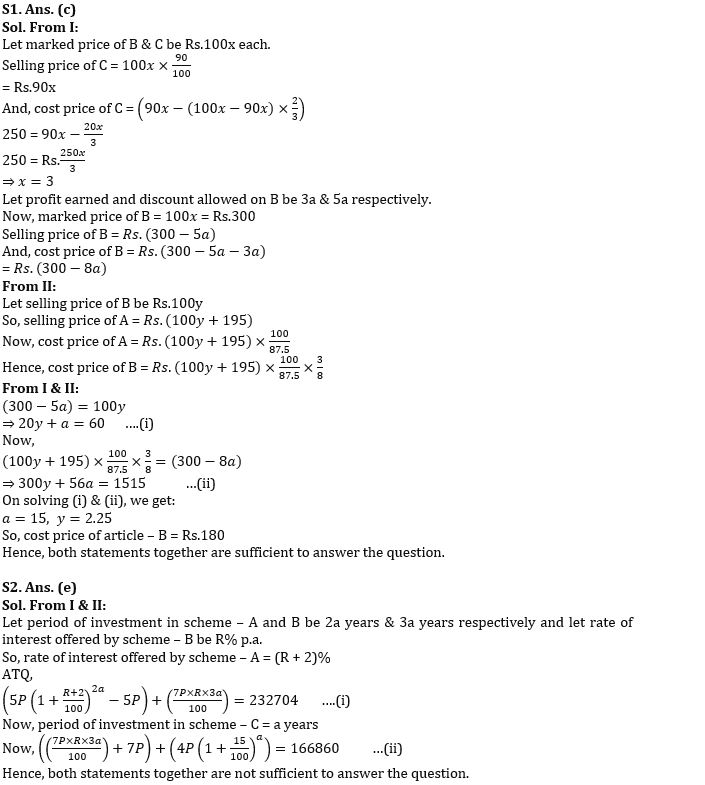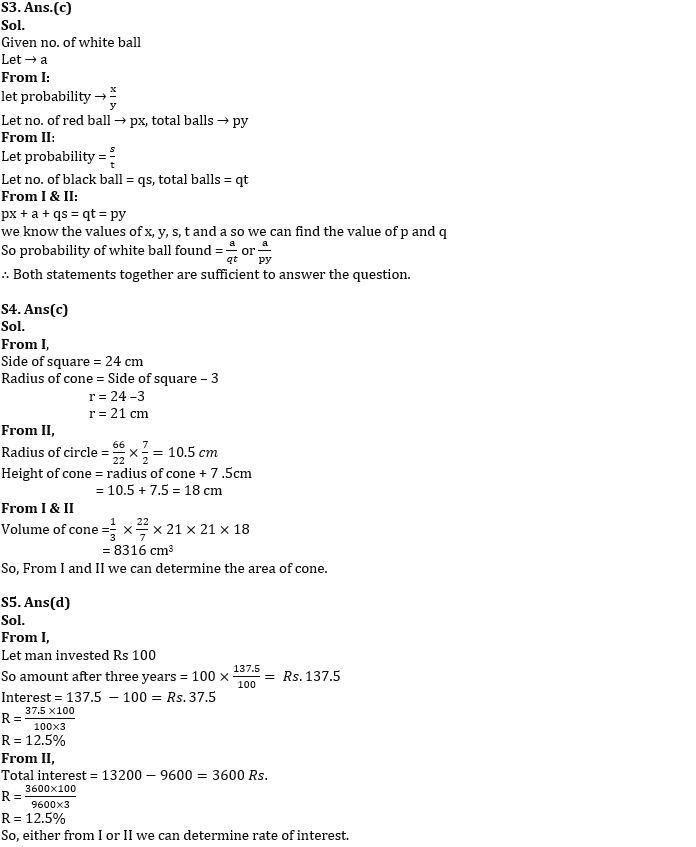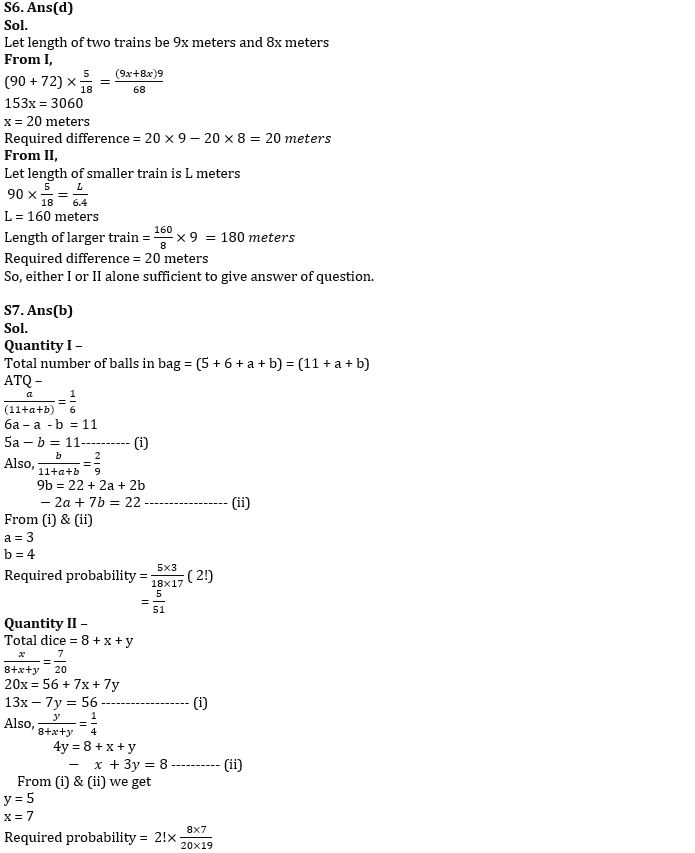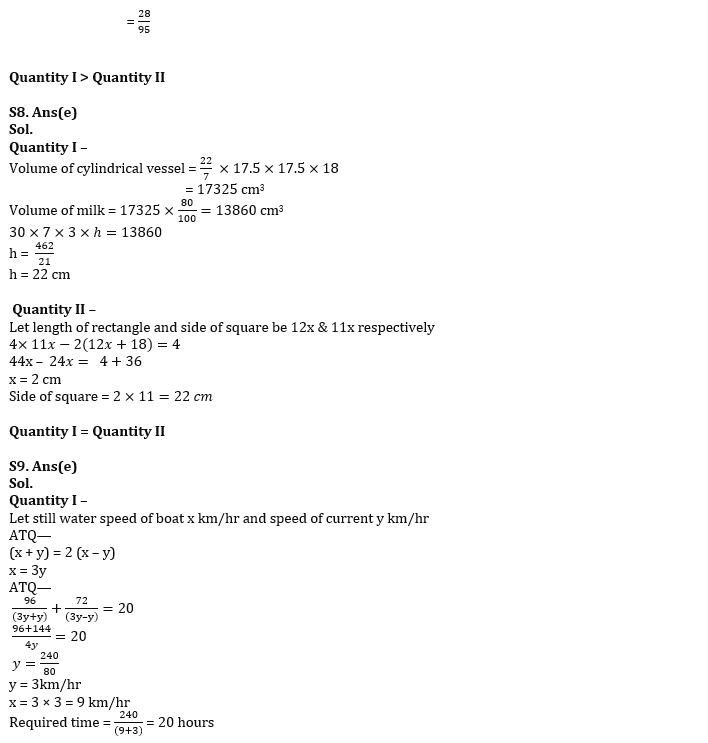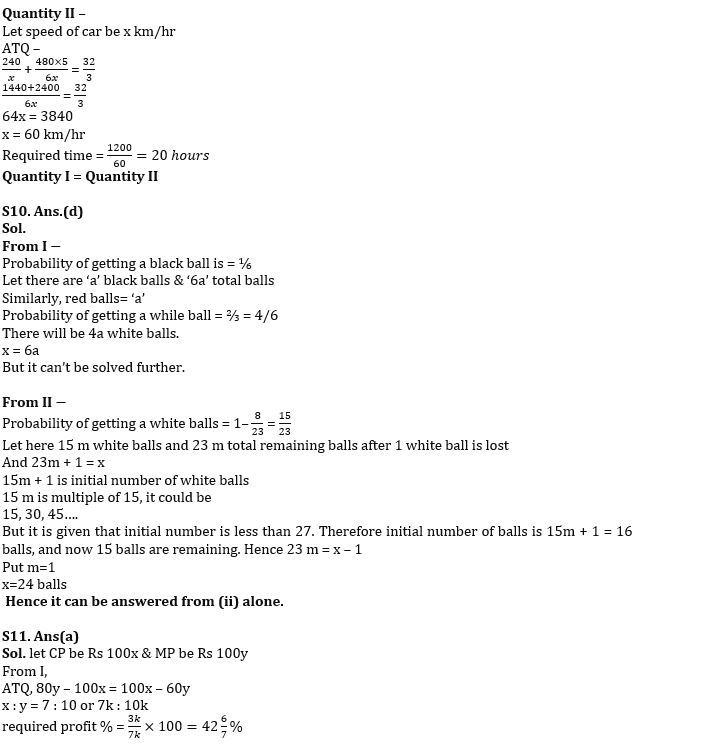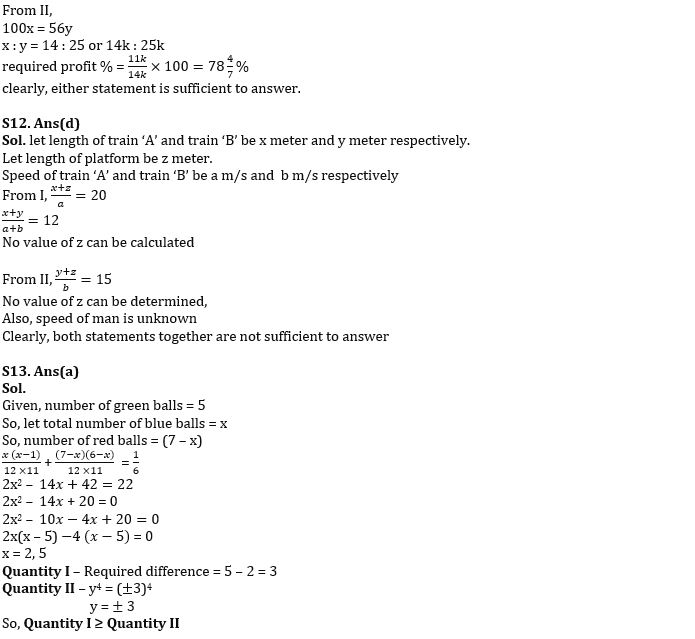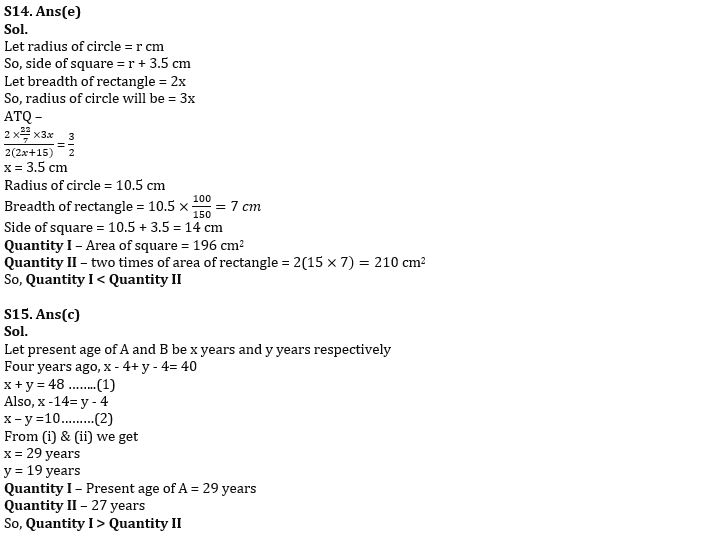## FAQs

#### Congratulations!